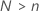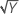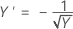# Methods and formulas for model information in Analyze Definitive Screening Design

## Design matrix

First, Minitab creates a design matrix from the factors and the model that you specify. The columns of this matrix represent the terms in the model. Then, Minitab adds additional columns for the constant term, blocks, and higher-order terms to complete the design matrix for the model in the analysis.

### Designs with all continuous factors

The form of the design matrix depends on whether a conference matrix exists with the same number of rows as factors. When this criterion is true, the columns of the design matrix that represent the factors have this form:
Where C is an n× n conference matrix with elements {-1, 0, 1} that satisfies this property:
When a conference matrix of the correct size does not exist, the columns that represent the factors are a subset of a larger conference matrix:
Where A is an N× n matrix with elements {-1, 0, 1} that satisfies this property:

The full design matrix contains columns besides the columns that represent factors. The design matrix contains a column of 1s for the constant term. The full design matrix also includes columns that represent any square or interaction terms in the model.

### Designs with categorical factors

For a design that includes categorical factors, Minitab replaces the single center point row in the design matrix with 2 pseudo center points. If the design has only 1 categorical factor, only two possible pseudo center points exist, so both points are in the design.

In the case where the design has more than 2 categorical factors, Minitab uses an iterative algorithm to select 2 pseudo center points to include. The algorithm seeks to minimize the variance of the regression coefficients for the linear effects in the model.

### Notation

TermDescription
CA conference matrix
0'A row of zeroes in a matrix that represent a center point run
Inthe n × n identity matrix
AA matrix that is a subset of a conference matrix with N rows and n columns whereNThe number of rows in the subset of the columns from the conference matrix
nThe number of factors in a design

## Coefficient (Coef)

In matrix terms, the formula that calculates the vector of coefficients in the model is:

TermDescription
Xdesign matrix
Yresponse vector

## Box-Cox transformation

Box-Cox transformation selects lambda values, as shown below, which minimize the residual sum of squares. The resulting transformation is Y λ when λ ≠ 0 and ln(Y) when λ = 0. When λ < 0, Minitab also multiplies the transformed response by −1 to maintain the order from the untransformed response.

Minitab searches for an optimal value between −2 and 2. Values that fall outside of this interval might not result in a better fit.

Here are some common transformations where Y′ is the transform of the data Y:

Lambda (λ) value Transformation
λ = 2 Y′ = Y 2
λ = 0.5 Y′ =λ = 0 Y′ = ln(Y )
λ = −0.5λ = −1 Y′ = −1 / Y

## Weighted regression

Weighted least squares regression is a method for dealing with observations that have nonconstant variances. If the variances are not constant, observations with:

• large variances should be given relatively small weights
• small variances should be given relatively large weights

The usual choice of weights is the inverse of pure error variance in the response.

The formula for the estimated coefficients is as follows:
This is equivalent to minimizing the weighted SS Error.

### Notation

TermDescription
Xdesign matrix
X'transpose of the design matrix
Wan n x n matrix with the weights on the diagonal
Yvector of response values
nnumber of observations
wiweight for the ith observation
yiresponse value for the ith observationfitted value for the ith observation
By using this site you agree to the use of cookies for analytics and personalized content.  Read our policy